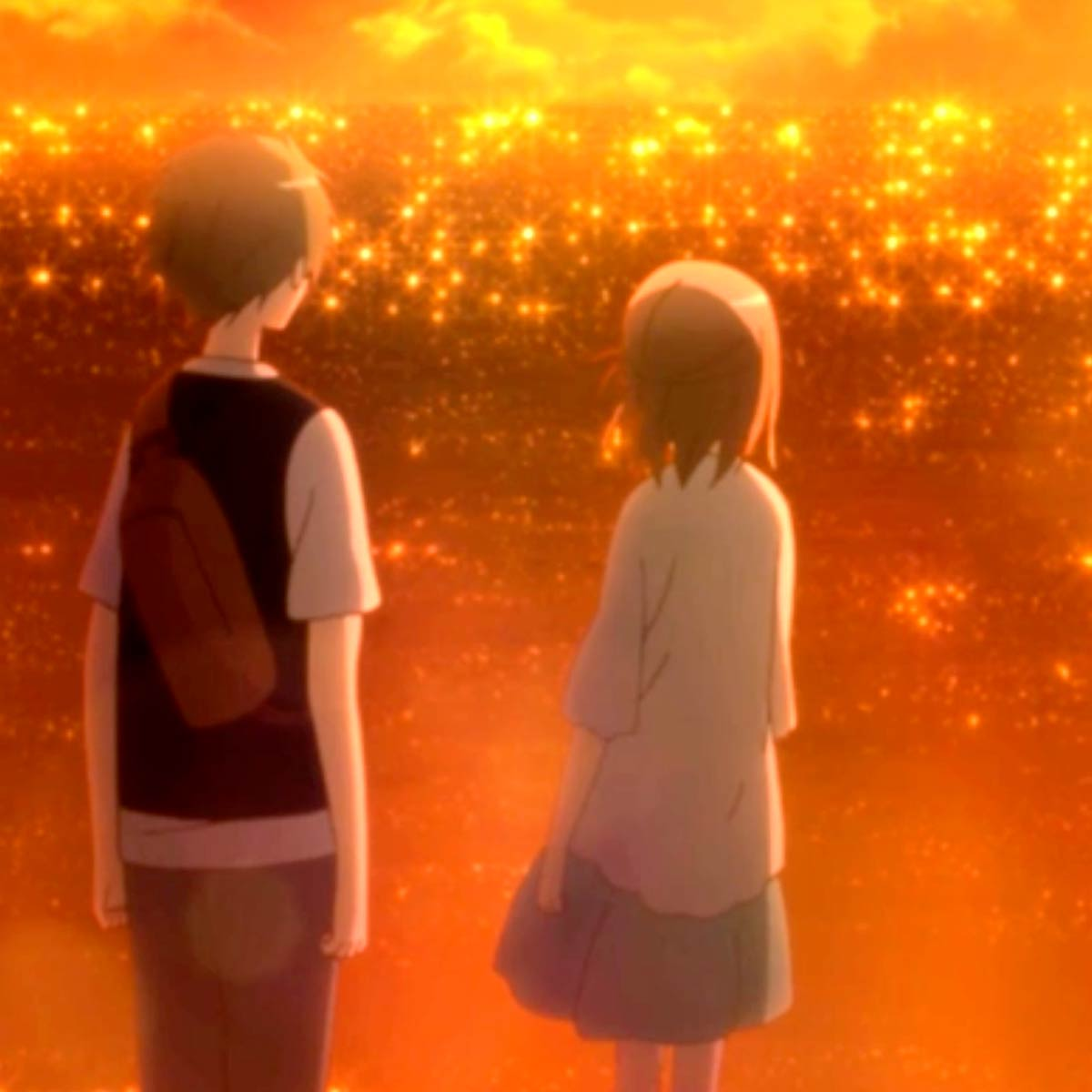# Tomohito Nishiura

2 Music Sheets

• ###### Birth Name:

Nishiura Tomohito

• ###### Born:

13 February 1973

• ###### Genres:

Tomohito Nishiura (西浦 智仁, Nishiura Tomohito) is a Japanese video game music composer. He works primarily on games developed by Level-5.

Credit: Wikipedia

## Artist's Music Sheets

•[zd] j [zd]x[gc] [vd] [xf] [dc] [xj] [zd] j [zd]x[lg] [md] [nf] [vg] h [zd]x[jc]x[dc]v[gc]x[db]c[xf]c[vd]c[xj]l[zd]x[jc]x[dc]v[gc]x[mdb]c[xnf]c[zvd]c[xj]l[zd]x[jc]x[dc]v[gc]x[db]c[xf]c[vd]c[xj]l[zd]x[jc]x[dc]v[gc]x[mdb]c[xnf]c[vd]c[xj]l[zd]x[jc]x[dc]v[gc]x[db]c[xf]c[vd]c[xj]l[zd]x[jc]x[dc]v[gc]x[mdb]c[xnf]c[vd]c[xj]l[dc]x[zj] d [gc]x[ld] fl[dc]x[zj] [dc]x[zj] d [gc]x[ld] fl[dc]x[zj] [dc]x[zj] d [gc]x[ld] fl[dc]x[zj] [dc]x[zj] d [gc]x[zy] [xd]d[fc]d[vg]h[d9]|[ed]fgh[j9]|o hg[f9] g[he] j[f9]|o|[d9]|[ed]fgl[k9]|o jh[j9] fg[he] f[g9]|o|[d9]|[ed]fgh[j9]|o hg[f9] g[he] j[f9]|o|[d9]|[ed]fgl[k9]|o jh[j9] fg[he] f[d9]|o|[c9]xz e cx[l9] l[oc]xz [c9]xz e cx[l9] l[oc]xz [c9]xz e cx[l9] l[oc]xz [c9]xz e cx[z92] [yx]y[uc]y[vi]o[zd]jzjzjzjzjzj[zd][jf][zg][jh][zd]jzjzjzjzjzj[zh][jg][zf][sj][zd]jzjzjzjzjzj[zd][jf][zg][jh][zd]jzjzjzjzjzj[zh][jg][zf][sj][zyd] [jd] [xd] [dc] [zd] [jd] [xd] [dc] [ztd] [jd] [xd] [dc] [zd] [jd] [xd] [dc] [zdE] [jd] [xd] [dc] [zd] [jd] [xd] [dc] [ztd] [jd] [xd] [dc] [zd] [jd] [xd] [dc] [zd] d d d d d [md] [vd] [zd] j [zd]x[gc] [vd] [xf] [dc] [xj] [zd] j [zd]x[lg] [md] [nf] [vg] h [zd]x[jc]x[dc]v[gc]x[db]c[xf]c[vd]c[xj]l[zd]x[jc]x[dc]v[gc]x[mdb]c[xnf]c[zvd]c[xj]l[zd]x[jc]x[dc]v[gc]x[db]c[xf]c[vd]c[xj]l[zd]x[jc]x[dc]v[gc]x[mdb]c[xnf]c[vd]c[xj]l[zd]x[jc]x[dc]v[gc]x[db]c[xf]c[vd]c[xj]l[zd]x[jc]x[dc]v[gc]x[mdb]c[xnf]c[vd]c[xj]l[dc]x[zj] d [gc]x[ld] fl[dc]x[zj] [dc]x[zj] d [gc]x[ld] fl[dc]x[zj] [dc]x[zj] d [gc]x[ld] fl[dc]x[zj] [dc]x[zj] d [gc]x[zy] [xd]d[fc]d[vg]h[d9]|[ed]fgh[j9]|o hg[f9] g[he] j[f9]|o|[d9]|[ed]fgl[k9]|o jh[j9] fg[he] f[g9]|o|[d9]|[ed]fgh[j9]|o hg[f9] g[he] j[f9]|o|[d9]|[ed]fgl[k9]|o jh[j9] fg[he] f[d9]|o|[c9]xz e cx[l9] l[oc]xz [c9]xz e cx[l9] l[oc]xz [c9]xz e cx[l9] l[oc]xz [c9]xz e cx[z92] [yx]y[uc]y[vi]o[zd]jzjzjzjzjzj[zd][jf][zg][jh][zd]jzjzjzjzjzj[zh][jg][zf][sj][zd]jzjzjzjzjzj[zd][jf][zg][jh][zd]jzjzjzjzjzj[zh][jg][zf][sj][zyd] [jd] [xd] [dc] [zd] [jd] [xd] [dc] [ztd] [jd] [xd] [dc] [zd] [jd] [xd] [dc] [zdE] [jd] [xd] [dc] [zd] [jd] [xd] [dc] [ztd] [jd] [xd] [dc] [zd] [jd] [xd] [dc] [zd] d d d d d [md] [vd]

Level: 5
Length: 02:12
Intermediate

#### Tomohito Nishiura

•Q ( W ( E ( Q ( q ( Q ( T ( E ( [YQ] ( W ( [IE] ( Q ( [qi] ( Q ( [TS] ( E ( [QP] ( W ( E ( T ( [tP] ( W ( r ( E|[YQ] ( W ( [IE] ( Q ( [qi] ( Q ( [T] ( ^ ( [QE] ( W ( E ( T ( [tE] ( W ( r ( [YE]iIO[QO] ( Q [P(] Q ( [QI] ( [qi] [a9] [qP] [P9] [qd] 9Pq [O9] [QP] ( W ( E ( T ( t [O(] [WI] [O(] [rI] (iE [Y(] [iQ] ( Q [I(] Q ( Q ( [qi] 9iqi9 [qi] [i9] qi9 [QI] [Y(] W ( E ( T ( t ( W ( r ( E ( [QP] ( Q [g(] Q [D(] Q [G(] [qg] ( Q [D(] T ( [SE]a[P(]O[QP] ( Q [g(] Q [D(] Q [G(] [qg] ( Q [H(] T ( E ( [SQ] (SQS(SQ ( [SQ] [S(] [qO] ( [QO] (OT (PE ( [QP] ( [QO]P[O(]I[iQ] (Yq [i(] [qI] ( Q [O(] T [S(] E [P(] [QP] ( W ( E ( T ( [tP] ( W ( r ( E ( [YQ] ( W ( [IE] ( Q ( [qi] ( Q ( [TS] ( E ( [QP] ( W ( E ( T ( [tP] ( W ( r ( E ( [YQ] ( W ( [IE] ( Q ( [qi] ( Q ( [T] ( ^ ( [QE] ( W ( E ( T ( [tE] ( W ( r ( E

Level: 4
Length: 01:12
Easy